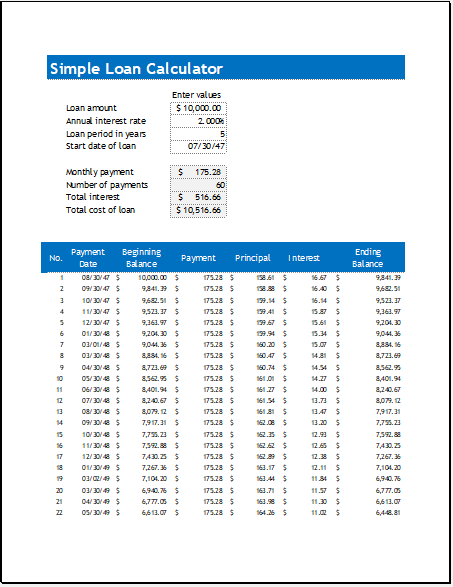# Simple Loan Calculator

All of us come across such a situation in which we run out of money and face different financial problems. In order to deal with all financial problems, many of us end up with the decision of borrowing the money. Whether we are planning to buy a new car or bringing improvements to our house, we can make the decision to borrow the money.

Borrowing money is not the only decision one has to make. Another important thing that every borrower is required to take into consideration is how much amount to be borrowed. The loan calculator is used in order to calculate the financial needs and the amount that should be borrowed to fulfill the financial needs in such a way that loan can be easily paid off through easy monthly payments.

## What is a simple loan calculator?

A loan calculator is a tool that is used to calculate the payments to be made each month. Using the loan calculator enables the user to calculate the secure and unsecured loan.

## When to use the loan calculator?

The loan calculator is used when a user wants to borrow the money. This calculator provides details about the loan. The user can use this calculator when he wants to know how long it will take to pay off the borrowed money and how much he will have to pay each month. Moreover, the loan calculator is also used when it is required to be determined whether to borrow the money or not. In other words, the loan calculator can be used to assess the financial needs of the person.

## What are the benefits of using the loan calculator?

1. When you want to figure out how the interest rate will impact your financial situation, you can use the loan calculator. There are different aspects of borrowing the money which is associated with complex mathematical calculations. A loan calculator is a great tool that makes it easier for the user to perform all the simple and complex calculations in no time.
2. All the information about the loan can be obtained by using the calculator depending on how many variables you want to add to the calculator such as the amount to be repaid, interest amount on the borrowed money, time period of the loan and a lot more.
3. Many people don’t realize the importance of calculating the loan and get themselves in the trouble later on when they realize that they have to pay the monthly payments which are more than expected. The loan calculator gives a clear and realistic picture of the situation so that the user knows everything about the money to be borrowed.

There are various empty fields in the loan calculator that ask the user to fill in so that the loan and several other details related to loan can be calculated. The main details provided to the loan calculator include total amount to be borrowed, terms of the loan in years, the interest rate per year and a lot more.

PreviewFormat: MS Excel [.xlsx]
File Size: 46 KB